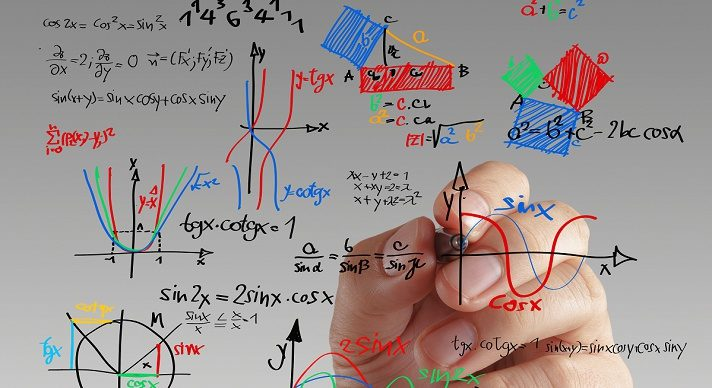# Algebra II### Course Overview

Algebra II builds upon the algebraic concepts taught in Algebra I, continuing on to functions, expressions, etc. and providing students with a more in-depth understanding of algebraic concepts.  It is taught by award-winning Acellus Master Teacher, Patrick Mara. Acellus Algebra II is A-G Approved through the University of California.

### Course Objectives & Student Learning Outcomes

In Acellus Algebra II, basic skills learned in Algebra I are reinforced and built upon. With the successful completion of this course, students will have the solid foundation in Algebra needed for continued success in more advanced math courses. Students will have reviewed expressions, equations, inequalities, and systems and extended their understanding of functions, equations, and graphs. They have attained a deeper understanding of linear, quadratic, exponential, and rational functions and how to transform them and use them to model situations. They also have a basic understanding of polynomial, radical, and logarithmic functions. Students have attained an understanding of complex numbers and know how to graph them and perform various mathematical operations with them. Students are experienced working with sequences and series. They are familiar with various conic sections, their graphs and equations. Students know how to perform operations on matrices and use them to solve systems of equations and to perform geometric transformations. Students are more confident with calculations involving permutations and combinations. They know how to calculate the probability associated with multiple events and also conditional probability and are familiar with probability models. They also have worked with binomial, normal, and probability distribution functions. Students are familiar with the unit circle and graphs of the sine, cosine, and tangent functions and how to translate them. They also know basic trig identities and how to use them to solve problems.This course was developed by the International Academy of Science. Learn More

### Scope and Sequence

Unit 1 – Algebraic Expressions In this unit students explore algebraic expressions, and sets of numbers.  They study solving equations and inequalities, simplifying and evaluating, and absolute value. Unit 2 – Functions In this unit students discuss the definition of a function, direct variation, slope-intercept, and point-slope.  They explore piece-wise defined functions, linear models, transformations of functions, absolute value functions, and inequalities in two variables. Unit 3 – Linear Systems In this unit students investigate solving graphically, substitution, elimination, and systems of inequalities.  They discuss linear programming, systems in three variables, and matrices. Unit 4 – Quadratic Functions In this unit students learn about transformations, standard form, quadratic regression, quadratic equations, quadratic formula, and quadratic inequalities.  They investigate factoring, completing the square, complex numbers, and graphing and representing operations of complex numbers. Unit 5 – Polynomials In this unit students study polynomial functions and equations, factored form, dividing polynomials, synthetic division, and finding roots.  They learn about the fundamental theorem of algebra, graphing polynomial functions, binomial theorem, and regression. Unit 6 – Radical Functions In this unit students explore the properties of exponents, rational exponents, adding radicals, and solving radical equations.  They study composition of functions, inverse functions, and graphing radical functions. Unit 7 – Exponential and Logarithmic Functions In this unit students discuss exponential and logarithmic functions and equations.  They explore properties of logarithms, and compound interest.  Following this unit students are presented with the Mid-Term Review and Exam. Unit 8 – Rational Fractions In this unit students investigate inverse variation, reciprocal functions, and graphs of rational functions.  They discuss adding, subtracting, multiplying, and dividing rational expressions, solving inequalities, and number theory. Unit 9 – Sequences and Series In this unit students learn about explicit versus recursive definitions.  They investigate arithmetic and geometric sequences and series. Unit 10 – Conic Sections In this unit students study definitions, parabolas, and circles.  They also learn about ellipses, hyperbola, and quadratic systems. Unit 11 – Probability and Statistics In this unit students explore permutations and combinations, probability, multiple events, conditional probability, and probability models.  They study analyzing data, standard deviation, samples and surveys, binomial and normal distributions, probability distribution functions, and expected value. Unit 12 – Matrices In this unit students discuss adding, subtracting, and multiplying matrices.  They explore inverses, solving systems using inverses, geometric transformations, and vectors. Unit 13 – Periodic Functions In this unit students investigate the definition of periodic, the unit circle, radian measure, and the sine, cosine, and tangent functions.   They discuss reciprocal and translating trigonometric functions. Unit 14 – Trig Identities and Equations In this unit students learn about basic identities, solving trig functions and inverse trig functions, right triangles, and the laws of sines and cosines.  They investigate sum and difference identities, and double and half angle identities.  Following this unit students are presented with the Final Review and Exam.